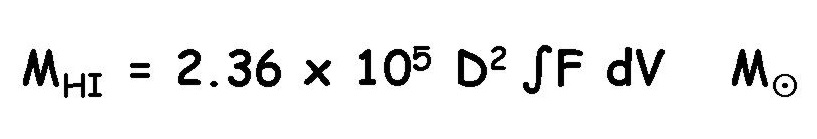## Deriving the total atomic hydrogen (HI) mass of a galaxy

ALFALFA detects the HI spectral profile of a galaxy, that is, its HI line flux over the frequency range which corresponds to the radial velocities of all the HI atoms in the galaxy. The total HI line flux, ∫F dV, in Jy-km/s, can be used to derive the total HI mass, in solar masses, using the distance, estimated from the systemic velocity via Hubble's law or by some other means.where D is the distance in Mpc.

This equation derives from the quantum mechanics of the hyperfine transition and the assumption that the HI gas is optically thin. If you want to know more about how the line arises and where that equation comes from, check out a couple of "classic" references:

Back to Using ALFA for ALFALFA
Back to Using ALFALFA for science

Last updated Wed Apr 23 08:30:53 EDT 2008 by martha